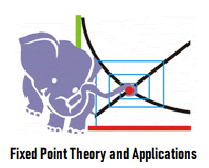# MTH604: Fixed Point Theory and Applications (Fall 2022)This course is intended as a brief introduction to the subject with a focus on Banach Fixed Point theorems fixed point theorem and its application to nonlinear differential equations, nonlinear integral equations, real and complex implicit functions theorems and system of nonlinear equations. Some generalizations and similar results e. g. Kannan Fixed Point theorems, Banach Fixed Point theorem for multi-valued mappings are also educated.

Basic concepts: metric spaces, complete metric spaces, Vector spaces (linear spaces) normed spaces, Banach spaces, Banachs contraction principle, non-expansive mappings and related fixed point theorems. Contractive maps, properties of fixed points set and minimal sets. Multi-valued mappings and related fixed point theorems. Best approximation theorems.

1. State intermediate value theorem.
2. State and prove the fixed point theorem.
3. Define attracting, repelling and neutral fixed points.
4. Consider a function $f(x)=x^2-1$. Find its fixed points and also find the nature of fixed point.
5. Define orbit of the point $x_0$ under function $f$.
6. Consider $C(x)=\cos (x)$. Draw the cobweb representation of the orbit of $-2$ under $C$.
7. Consider $C(x)=\cos (x)$. Draw the cobweb representation of the orbit of $0$ under $C$.
8. Let $L(x)=-1.4x$. Draw the cobweb representation of the orbit of $0.1$ under $L$.
9. Consider a function $L(x)=mx$, where $m\in \mathbb{R}$. Find the value of $m$ for which $x=0$ is attracting fixed point.
10. Let $F(x)=2(\sin x)^2$. Find the interval in which $F$ has attracting fixed point.
11. Prove that $\frac{1}{2}e^x \cos(x)$ has attracting fixed point in the interval $(-\infty,0]$.
12. State the mean value theorem.
13. State and prove attracting fixed point theorem.
14. State and prove repelling fixed point theorem.
15. Find the fixed point of $f(x)=2x-2x^2$ by iteration method by taking initial guess $x_0=0.1$.
16. Let $B(x;r)$ represents open ball with centre $x$ and radius $r$ in some metric space. Find $B\left(0;5 \right)$ in discrete metric on $\mathbb{R}$.
17. Let $B(x;r)$ represents open ball with centre $x$ and radius $r$ in some metric space. Find $B\left(1;0.7 \right)$ in discrete metric on $\mathbb{R}$.
18. Let $B(x;r)$ represents open ball with centre $x$ and radius $r$ in some metric space. Find $B\left(2;5 \right)$ in usual metric on $\mathbb{R}$.
19. Define: Lipschtiz continuous.
20. Define contraction map.
21. Define contractive map.
22. Define nonexpansive map.
23. Prove that every Lipschtiz continuous is continuous.
24. Prove that every contractive map is continuous.
25. Prove that every contraction is continuous.
26. State and prove Banach contraction principle.
27. Let $(X,d)$ be a metric space and $F:X\to X$ be a contration, then prove that $\{ F^n(x)\}$ is Cauchy sequence.
28. Let $(X,d)$ be a metric space and $F:X\to X$ be a contration, then prove that $\{d(F^m(x),F^n(x) \leq \frac{L^n}{1-L}d(x,F(x) \}$ for $m>n$, $n\in \{1,2,...\}$.
29. Define compact metric space.
30. Let $(X,d)$ be a compact metric space and $T:X\to X$ be a contractive mapping. Then prove that $T$ has unique fixed point.
31. Let $(X, d)$ be a complete metric space and let $B\left(x_0, r\right)$ be open ball with centre at $x_0 \in X$ and radius $r>0$. Suppose $F: B\left(x_0, r\right) \rightarrow X$ is a contraction with $d\left(F\left(x_0\right), x_0\right)<(1-L) r$. Then prove that $F$ has a unique fixed point in $B\left(x_0, r\right)$.
32. Sketch the statement of above theorem.
33. Let $(X, d)$ be a complete metric space and let $B\left(x_0, r\right)$ be open ball with centre at $x_0 \in X$ and radius $r>0$. Suppose $F: B\left(x_0, r\right) \rightarrow X$ is a contraction with $d\left(F\left(x_0\right), x_0\right)<(1-L) r$. Then prove that $F:\overline{B(x_0,r_0)} \to \overline{B(x_0,r_0)}$ for $0<r_0<r$.
34. Let $(X,d)$ be a complete metric space and let $B(x_0,r)$ be open ball with center $x_0\in X$ and $r>0$. Suppose $F: B(x_0,r)\to X$ is a contraction with Lipschitz constant $L$ and also $d(F(x_0),x_0)<(1-L)r$. Then prove that $\overline{B(x_0, r_0)} \subset B(x_0, r)$ for $0<r_0<r$.
35. Let $\overline{B}_r$ be the closed ball of radius $r > 0$, centred at zero, in a Banach space $E$ with $F : \overline{B}_r \to E$ a contraction and $F (\partial\overline{B}_r) \subset \overline{B}_r$. Then prove that $F$ has a unique fixed point in $\overline{B}_r$.
36. Sketch the statement of above theorem.
37. Prove that $G$ is contraction, where $G(x)=\frac{x+F(x)}{2}$, on $X$ if $F$ is contraction on $X$.
38. Let $\overline{B}_r$ be the closed ball of radius $r > 0$, centred at zero, in a Banach space $E$ with $F : \overline{B}_r \to E$ a contraction and $F (\partial\overline{B}_r) \subset \overline{B}_r$. Then prove that $G: \overline{B}_r \to \overline{B}_r$, where $G(x)=\frac{x+F(x)}{2}$.

Notes and videos can be downloaded from the following university official page:

1. B. Bollobas: W. Fulton, A. Katok, F. Kirwan and P. Sarnak: Fixed Point Theory and Applications, Cambridge University Press, 2001.
2. K. Geoble and W.A Kirk: Topics in Metric Fixed Theory, Cambridge university Press, 1990
3. M.C. Joshi And R.K. Bose: Some Topics in Nonlinear Functional Analysis, John Wiley and Sons, 1985
4. James Dugundji and A. Granas: Fixed Point Theory, Vol. 1, Polish Scientific Publishers, 1982
5. W.A. Kirk and B. Sims: Handbook of Metric Fixed Point Theory, Klawer Academic Publishers 2001
6. M. Aslam Noor, Principles of Variational Inequalities Lapt-Lambert Academic Publishing AG & Co. Saarbrucken, Germany 2009.